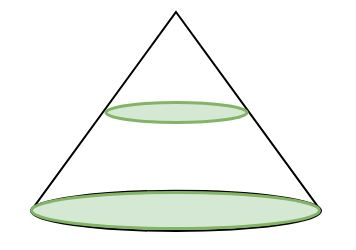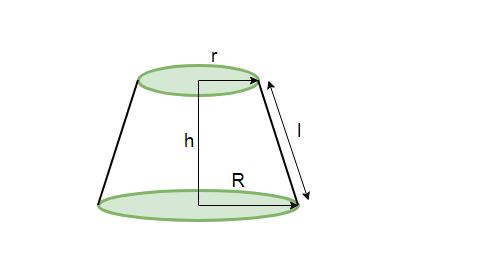# Program for Volume and Surface area of Frustum of Cone

Given slant height, height and radius of a frustum of a cone, we have to calculate the volume and surface area of the frustum of a cone.

Frustum of cone
In geometry, a frustum is the portion of a solid (normally a cone or pyramid) that lies between one or two parallel planes cutting it.
If we cut a right circular cone by a plane parallel to its base, the portion of the solid between this plane and the base is known as the frustum of a cone.

Given below is a right circular cone.The right circular cone after being cut by a plane parallel to its base results in a frustum as follows:which has a circular base at bottom of radius R
circular upper portion with radius r
height h
and slant height l

• Volume of frustum of cone:
• ```Volume (V) = 1/3 * pi * h(r2 + R2 + r*R)

where
r = radius of smaller circle
R = radius of bigger circle (or radius of base of the cone)
h = height of the frustum
```
• Curved Surface Area of frustum of cone:
• ```Curved Surface Area (CSA) = pi * l(R + r)

where
r = radius of smaller circle
R = radius of bigger circle
l = slant height of the frustum
```
• TotalSurface Area of frustum of cone:
• ```Total Surface Area (TSA) = pi * l(R + r) + pi(R2 + r2)

where
r = radius of smaller circle
R = radius of bigger circle
l = slant height of frustum
```

Examples:

```Input : Radius of smaller circle = 3
Radius of bigger circle = 8
Height of frustum = 12
Slant height of frustum = 13
Output :
Volume Of Frustum of Cone : 1218.937
Curved Surface Area Of Frustum of Cone : 449.24738
Total Surface Area Of Frustum of Cone : 678.58344

Input : Radius of smaller circle = 7
Radius of bigger circle = 10
Height of frustum = 4
Slant height of frustum = 5

Output :
Volume Of Frustum of Cone : 917.34436
Curved Surface Area Of Frustum of Cone : 267.03516
Total Surface Area Of Frustum of Cone : 735.1321
```

## C++

 `// CPP program to calculate Volume and ` `// Surface area of frustum of cone ` `#include ` `using` `namespace` `std; ` ` `  `float` `pi = 3.14159; ` ` `  `// Function to calculate Volume of frustum of cone ` `float` `volume(``float` `r, ``float` `R, ``float` `h) ` `{ ` `    ``return` `(``float``(1) / ``float``(3)) * pi * h * ` `                    ``(r * r + R * R + r * R); ` `} ` ` `  `// Function to calculate Curved Surface area of ` `// frustum of cone ` `float` `curved_surface_area(``float` `r, ``float` `R, ``float` `l) ` `{ ` `    ``return` `pi * l * (R + r); ` `} ` ` `  `// Function to calculate Total Surface area of  ` `// frustum of cone ` `float` `total_surface_area(``float` `r, ``float` `R, ``float` `l,  ` `                                           ``float` `h) ` `{ ` `    ``return` `pi * l * (R + r) + pi * (r * r + R * R); ` `} ` ` `  `// Driver function ` `int` `main() ` `{ ` `    ``float` `small_radius = 3; ` `    ``float` `big_radius = 8; ` `    ``float` `slant_height = 13; ` `    ``float` `height = 12; ` ` `  `    ``// Printing value of volume and surface area ` `    ``cout << ``"Volume Of Frustum of Cone : "` `         ``<< volume(small_radius, big_radius, height)  ` `         ``<< endl; ` ` `  `    ``cout << ``"Curved Surface Area Of Frustum of Cone : "` `         ``<< curved_surface_area(small_radius, big_radius,  ` `                                 ``slant_height) << endl; ` ` `  `    ``cout << ``"Total Surface Area Of Frustum of Cone : "` `         ``<< total_surface_area(small_radius, big_radius,  ` `                                 ``slant_height, height); ` `    ``return` `0; ` `} `

## Java

 `// Java program to calculate Volume and Surface area ` `// of frustum of cone ` ` `  `public` `class` `demo { ` ` `  `    ``static` `float` `pi = ``3``.14159f; ` ` `  `    ``// Function to calculate Volume of frustum of cone ` `    ``public` `static` `float` `volume(``float` `r, ``float` `R, ``float` `h) ` `    ``{ ` `        ``return` `(``float``)``1` `/ ``3` `* pi * h * (r * r + R * R + ` `                                                ``r * R); ` `    ``} ` ` `  `    ``// Function to calculate Curved Surface area of ` `    ``// frustum of cone ` `    ``public` `static` `float` `curved_surface_area(``float` `r,  ` `                                   ``float` `R, ``float` `l) ` `    ``{ ` `        ``return` `pi * l * (R + r); ` `    ``} ` ` `  `    ``// Function to calculate Total Surface area of  ` `    ``// frustum of cone ` `    ``public` `static` `float` `total_surface_area(``float` `r,  ` `                         ``float` `R, ``float` `l, ``float` `h) ` `    ``{ ` `        ``return` `pi * l * (R + r) + pi * (r * r + R * R); ` `    ``} ` ` `  `    ``// Driver function ` `    ``public` `static` `void` `main(String args[]) ` `    ``{ ` `        ``float` `small_radius = ``3``; ` `        ``float` `big_radius = ``8``; ` `        ``float` `slant_height = ``13``; ` `        ``float` `height = ``12``; ` ` `  `    ``// Printing value of volume and surface area ` `        ``System.out.print(``"Volume Of Frustum of Cone : "``); ` `        ``System.out.println(volume(small_radius,  ` `                            ``big_radius, height)); ` ` `  `        ``System.out.print(``"Curved Surface Area Of"` `+  ` `                            ``" Frustum of Cone : "``); ` `        ``System.out.println(curved_surface_area(small_radius, ` `                                  ``big_radius, slant_height)); ` `        ``System.out.print(``"Total Surface Area Of"` `+  ` `                ``" Frustum of Cone : "``); ` ` `  `        ``System.out.println(total_surface_area(small_radius,  ` `                        ``big_radius, slant_height, height)); ` `    ``} ` `} `

## Python3

 `# Python3 code to calculate  ` `# Volume and Surface area of ` `# frustum of cone ` `import` `math ` ` `  `pi ``=` `math.pi ` ` `  `# Function to calculate Volume ` `# of frustum of cone ` `def` `volume( r , R , h ): ` `    ``return` `1` `/``3` `*` `pi ``*` `h ``*` `(r  ` `            ``*` `r ``+` `R ``*` `R ``+` `r ``*` `R) ` ` `  `# Function to calculate Curved  ` `# Surface area of frustum of cone ` `def` `curved_surface_area( r , R , l ): ` `    ``return` `pi ``*` `l ``*` `(R ``+` `r) ` ` `  `# Function to calculate Total   ` `# Surface area of frustum of cone ` `def` `total_surface_area( r , R , l , h ): ` `    ``return` `pi ``*` `l ``*` `(R ``+` `r) ``+` `pi ``*` `(r ` `                            ``*` `r ``+` `R ``*` `R) ` `     `  `# Driver Code ` `small_radius ``=` `3` `big_radius ``=` `8` `slant_height ``=` `13` `height ``=` `12` ` `  `# Printing value of volume  ` `# and surface area ` `print``(``"Volume Of Frustum of Cone : "` `                                ``,end``=``'') ` `print``(volume(small_radius, big_radius, ` `                                ``height)) ` ` `  `print``(``"Curved Surface Area Of Frustum"``+` `                    ``" of Cone : "``,end``=``'') ` `print``(curved_surface_area(small_radius, ` `                ``big_radius,slant_height)) ` ` `  `print``(``"Total Surface Area Of Frustum"``+` `                    ``" of Cone : "``,end``=``'') ` `print``(total_surface_area(small_radius,  ` `        ``big_radius,slant_height, height)) ` ` `  `# This code is contributed by "Sharad_Bhardwaj". `

## C#

 `// C# program to calculate Volume and  ` `// Surface area of frustum of cone ` `using` `System; ` ` `  `public` `class` `demo { ` ` `  `    ``static` `float` `pi = 3.14159f; ` ` `  `    ``// Function to calculate  ` `    ``// Volume of frustum of cone ` `    ``public` `static` `float` `volume(``float` `r, ``float` `R, ``float` `h) ` `    ``{ ` `        ``return` `(``float``)1 / 3 * pi * h * (r * r + R *  ` `                                        ``R + r * R); ` `    ``} ` ` `  `    ``// Function to calculate Curved ` `    ``// Surface area of frustum of cone ` `    ``public` `static` `float` `curved_surface_area(``float` `r,  ` `                                ``float` `R, ``float` `l) ` `    ``{ ` `        ``return` `pi * l * (R + r); ` `    ``} ` ` `  `    ``// Function to calculate Total ` `    ``// Surface area of frustum of cone ` `    ``public` `static` `float` `total_surface_area(``float` `r, ``float` `R,  ` `                                           ``float` `l, ``float` `h) ` `    ``{ ` `        ``return` `pi * l * (R + r) + pi * ` `                    ``(r * r + R * R); ` `    ``} ` ` `  `    ``// Driver function ` `    ``public` `static` `void` `Main() ` `    ``{ ` `        ``float` `small_radius = 3; ` `        ``float` `big_radius = 8; ` `        ``float` `slant_height = 13; ` `        ``float` `height = 12; ` ` `  `    ``// Printing value of volume  ` `    ``// and surface area ` `    ``Console.Write(``"Volume Of Frustum of Cone : "``); ` `     `  `    ``Console.WriteLine(volume(small_radius,  ` `                    ``big_radius, height)); ` ` `  `    ``Console.Write(``"Curved Surface Area Of"` `+  ` `                     ``" Frustum of Cone : "``); ` `                      `  `    ``Console.WriteLine(curved_surface_area(small_radius, ` `                            ``big_radius, slant_height)); ` `                             `  `    ``Console.Write(``"Total Surface Area Of"` `+  ` `                    ``" Frustum of Cone : "``); ` ` `  `    ``Console.WriteLine(total_surface_area(small_radius,  ` `                    ``big_radius, slant_height, height)); ` `    ``} ` `} ` ` `  `// This article is contributed by vt_m `

## PHP

 ` `

Output:

```Volume Of Frustum of Cone : 1218.937
Curved Surface Area Of Frustum of Cone : 449.24738
Total Surface Area Of Frustum of Cone : 678.58344
```

Attention reader! Don’t stop learning now. Get hold of all the important DSA concepts with the DSA Self Paced Course at a student-friendly price and become industry ready.

My Personal Notes arrow_drop_upCheck out this Author's contributed articles.

If you like GeeksforGeeks and would like to contribute, you can also write an article using contribute.geeksforgeeks.org or mail your article to contribute@geeksforgeeks.org. See your article appearing on the GeeksforGeeks main page and help other Geeks.

Please Improve this article if you find anything incorrect by clicking on the "Improve Article" button below.

Improved By : vt_m